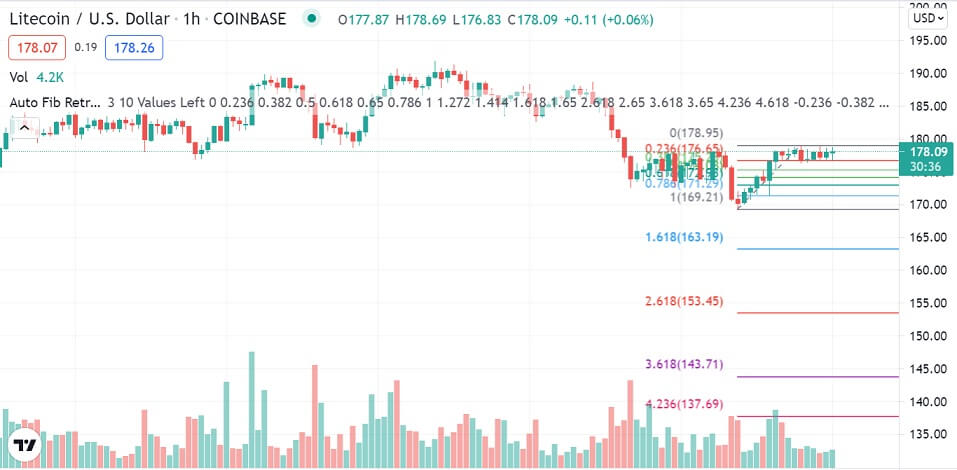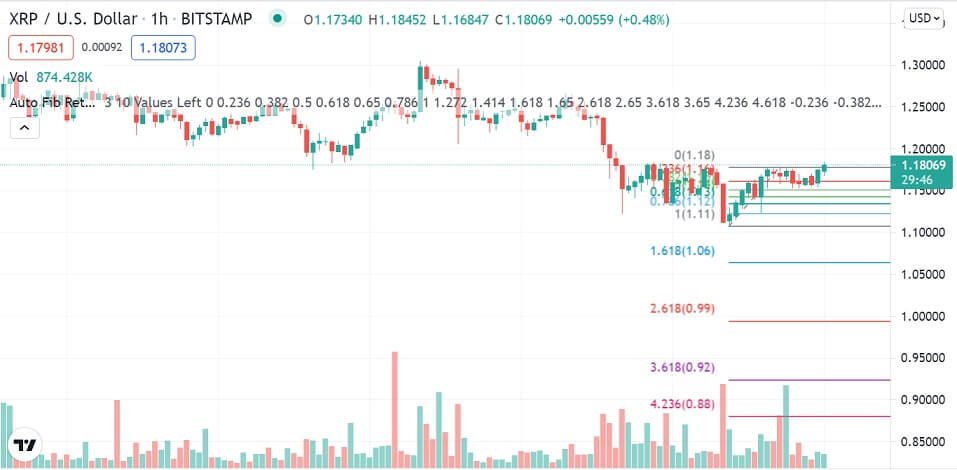Ethereum, Litecoin, & Ripple’s XRP Technical Analysis – 26th August

Bulls take control after a volatile day, Wednesday

Last Updated August 26th 2021

Ethereum

Ethereum had a mixed day on Wednesday and ended the day pretty much where it started.

It started the day range-bound between the 38.2% Fibonacci resistance at \$3217.43 and the 50.0% Fibonacci support at \$3168.23.

However, by mid-morning, bears took control and pushed it through the 50.0% Fibonacci support and with high volumes.

The selloff was so strong that Ethereum even pushed through the 61.8% Fibonacci support at \$3118.08.

However, by mid-day, bullish momentum was on the rise in the broader market. What followed is that Ethereum made a V-Shaped recovery.

It rallied through the 61.8% Fibonacci, now intra-day resistance, and the 50.0% Fibonacci resistance at \$3168.23.

By the end of the day, Ethereum was trading at the 38.2% Fibonacci resistance at \$3217.43.

Ethereum is currently trading at \$3218.08 and was up by 0.18% at the time of writing. It started the day trading at the 38.2% Fibonacci resistance and was oscillating around it when going to press.A glance at the day ahead

In the day, the critical level to watch will be the 38.2% Fibonacci resistance at \$3217.43.

If bulls are strong enough to push Ethereum through the 38.2% Fibonacci, and with high volumes, the next key level to watch would be the 23.6% Fibonacci at \$3277.99.

If bulls can push Ethereum through this level, then prices above \$3300 could be possible in the day.

On the other hand, if the 38.2% Fibonacci resistance holds and bears take control, the key level to watch would be the 50.0% Fibonacci support at \$3168.23.

If bears are strong enough to push Ethereum through this support, the next key level to watch would be the 61.8% Fibonacci support at \$3118.08. If it’s broken, then prices below \$3000 could be possible in the day.

However, if volumes decline in the day, Ethereum could trade in a range around the 38.2% Fibonacci resistance at \$3217.43.

A glance at the technicals

Key resistance: 38.2% Fibonacci at \$3217.43

Key support: 50.0% Fibonacci at \$3168.23

Litecoin

Litecoin had a mixed day on Wednesday and ended the day where it started.

It started the day range-bound between the 50.0% Fibonacci resistance at \$177.08 and the 61.8% Fibonacci support at \$173.08.

However, by mid-morning, bears took control and pushed it through the 61.8% Fibonacci support, and with high volumes.

The selloff that ensued saw Litecoin test lows of \$169.00 by midday.  However, in the early afternoon, bullish momentum was on the rise in the broader market.

What followed is that Litecoin made a V-Shaped recovery. It rallied through the 61.8% Fibonacci, now intra-day resistance at \$173.65, and with high volumes.

By late afternoon, Litecoin was trading on the 50.0% Fibonacci resistance at \$177.08. It oscillated around this resistance all through Wednesday evening.

Litecoin is currently trading at \$178.51 and was up by 2.14% at the time of writing. It started the day bullish and broke through the 50.0% Fibonacci resistance in the first three hours of the day. At the time of going to press, the 50.0% Fibonacci was now the day’s support.A glance at the day ahead

In the day, the key level to watch will be the 38.2% Fibonacci resistance at \$180.51.

If bulls are strong enough to push Litecoin through the 38.2% Fibonacci, and with high volumes, the next key level to watch would be the 23.6% Fibonacci at \$184.78.

If bulls push Litecoin through this level, then prices above \$190 could be possible in the day.

On the other hand, if the 38.2% Fibonacci resistance holds and bears take control, the key level to watch would be the 50.0% Fibonacci support at \$177.08.

If bears are strong enough to push Litecoin through this support, the next key level to watch would be the 61.8% Fibonacci support at \$173.65. If it’s broken, then prices below \$170 could be possible in the day.

However, if volumes decline in the day, then Litecoin could trade in a range between the 38.2% Fibonacci resistance at \$180.51 and the 50.0% Fibonacci support at \$177.08.

A glance at the technicals

Key resistance: 38.2% Fibonacci at \$180.51

Key support: 50.0% Fibonacci at \$177.08

Ripple’s XRP

Ripple’s XRP had a mixed, albeit low volumes day, on Wednesday. By the end of the day, it was trading pretty much where it started.

XRP started the day range-bound between the 50.0% Fibonacci resistance at \$1.17851 and the 61.8% Fibonacci support at \$1.14829.

However, by mid-morning, bears took control and pushed it through the 61.8% Fibonacci support, and with high volumes.

Just before midday, XRP dipped to a low of \$1.11109.  However, shortly after, bullish momentum was on the rise in the broader market. What followed is that XRP made a V-Shaped recovery.

It rallied through the 61.8% Fibonacci intra-day resistance at the time.  By the end of the day, XRP was trading at the 50.0% Fibonacci resistance at \$1.17851.

XRP is currently trading at \$1.17 and is up by 2.05%. It started the day trading between the 50.0% Fibonacci resistance at \$1.17851 and the 61.8% Fibonacci support at \$1.14829.A glance at the day ahead

In the day, the key levels to watch will be the 50.0% Fibonacci resistance at \$1.17851 and the 61.8% Fibonacci support at \$1.14829.

If bulls are strong enough to push XRP through the 50.0% Fibonacci resistance and with high volumes, the next key level to watch would be the 38.2% Fibonacci at \$1.20807.

If bulls can push XRP through this level, then prices above \$1.23 could be possible in the day.

On the other hand, if the 50.0% Fibonacci resistance holds, and bears take control, the key level to watch would be the 61.8% Fibonacci support at \$1.14829.

If bears are strong enough to push XRP through this support, the next key level to watch would be Wednesday’s low of \$1.10799. If it’s broken, then prices below \$1.05 could be possible in the day.

However, if volumes decline in the day, then XRP could trade in a range between the 50.0% Fibonacci resistance at \$1.17851 and the 61.8% Fibonacci support at \$1.14829.

A glance at the technicals

Key resistance: 50.0% Fibonacci at \$1.17851

Key support: 61.8% Fibonacci at \$1.14829